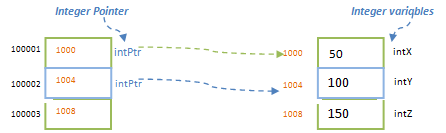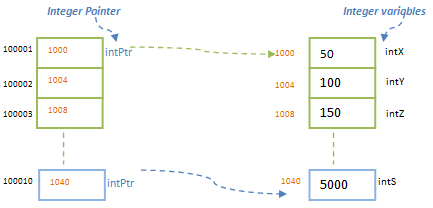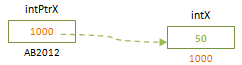Pointer Arithmetic in C Programming

As we have seen earlier, even though pointer is a variable and points to different data values, it holds the memory address of the variables. These memory addresses are in hexadecimal form and are one type of numerals. Hence we can perform arithmetic calculations on memory address and hence on the pointers. We have four basic arithmetic operations allowed to perform on pointers – incrementing (‘+’ and ‘++’) and decrementing (‘-‘ and ‘—‘). These are the unary operators used to increment and decrement  addresses stored in the pointers.

Identifiers in C Programming

intPtr++; // increments the value of pointer and assigns the new value to intPtr itselfNote here we have not used ‘*’ while incrementing. Since we are incrementing the address that  a pointer is holding, only pointer variable name is used. If we use ‘*’ along with pointer variable name, then it will point to the value that it is pointing to, and that value will be incremented (it will be an error if it is character pointer and ‘*’ is used!). Also note that it is not incrementing its own address, but the value stored in it. Its own address may or may not be contiguous address as shown above. But the values (address of variable that it is pointing to) are incremented serially according to datatype.

Similarly we can decrement the address of the pointers using ‘—‘ operator. It decrements the address in the same way as it increments the address of a pointer. That means, if the pointer is a character pointer, then it decrements the address by 1 byte; if it is an integer pointer then it decrements it by 4 bytes and so on.

intPtr–;

We can pre-increment/ pre-decrement the pointer variable or post-increment / post-decrement the pointer variable. This works in the same way as any normal variable is pre / post incremented or decremented. But only difference is that number of bytes incremented or decremented depends on the datatype of the pointer variable. Hence ++intPtr and intPtr++ will have same values after its execution. But first one will increment the address of pointer variable and then use that address value in the code. While the second one will use the current address first and then increment the address value. Similarly,  –intPtr and intPtr—have the same result except the way the compiler evaluates it.

C Inputs and Outputs in C Programming

The unary operators ‘++’ and ‘—‘ are used to increment or decrement the address to next location in the memory. What we will do if we need to move pointer address by 10? We may need to use ‘++’ 10 times in the code! Imagine the length and complexity of the code if this number is very big! Hence C provides us two other unary operator ‘+’ and ‘-‘ to increment and decrement. Using these operators, we can specify how much needs to be incremented or decremented.

Suppose we need to increment an integer pointer intPtr by 10. Then we specify it as below :

intPtr = intPtr +10;

OR
intPtr+ = 10; // it is same as above line of codeHere also, when we increment by 10, it will move the address by 40 bytes as integer occupies 4 bytes each. Hence after incrementing the pointer by 10, pointer variable will move to address 1040, which is an address of another variable. Please note difference between the values of address of pointer variable (100001, 100002 etc) and address of variable (1000, 1004 etc) that it contains while incrementing. This will clear explain how pointer arithmetic works. This is one of the ways to make the pointer to point to different variables of same type.

In the same way as increment above, we can use ‘-‘ operator to decrement the address. It decrements the address by checking the datatype of the pointer variable.

intPtr = intPtr -10;

OR
intPtr- = 10; // it is same as above line of code

This concept of pointer arithmetic lead to use pointers for arrays. Let us see them in detail below.
In addition to incrementing and decrementing the pointers, we can even compare them. We use comparison operators ‘==’, ‘<’, ‘<=’, ‘>’ and ‘>=’ to compare pointers. In this case we can either compare the address that the pointer is pointing to or the values of the variable that a pointer is pointing to (by using ‘*’ before pointer variable).

Register Storage Class in C Programming

Suppose intX is a variable and intPtrX is a pointer variable pointing to intX. Suppose intX has value 50 and its address is 1000.Now if we want to compare the address of intX and intPtrX, then we compare it as below:

intPtrX == &intX; // checks if addresses are equal, i.e.; 1000 == 1000
intPtrX <= &intX; // checks if address of intPtrX is less than or equal to intX address

Please note the difference between the notation of integer variable and pointer variable to get address values. Pointer variable is used without ‘*’ or ‘&’ as we need to get the address stored in it. But integer variable is used with ‘&’ as we need to compare its address with the pointer variable.

Which addresses will be compared in below case?

&intPtrX == &intX; // checks if addresses of pointer variable and integer variables are equal

Above line of code will compare address of pointer variable, AB2012 with the address of integer variable, 1000. Hence we need to be careful while using the pointers and their operators.